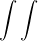# Use the given transformation to evaluate the integral

### Question:

Use the given transformation to evaluate the integral.

u= x+y, v= -2x+y;

R5y dxdy where R is the parallelogram bounded by the lines y= -x+1, y=-x+4, y= 2x+2, y= 2x+5

a. 85/4

b. 65/4

c.85/2

d.65/2